# Evaluation of IntegralsIt is helpful to define the new magnetic flux-surface label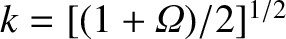. (See Section 8.11.) It follows from Equation (11.101) that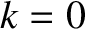at the O-points of the magnetic island chain, and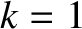on the magnetic separatrix. Hence, we can write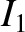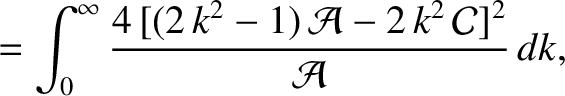(11.155)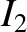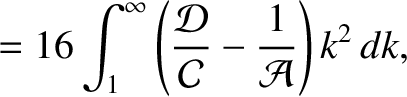(11.156)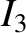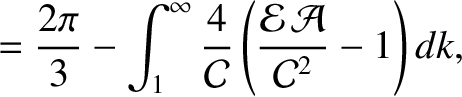(11.157)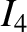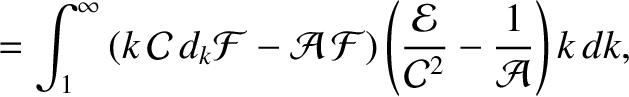(11.158)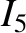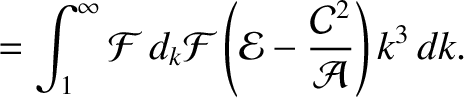(11.159)

where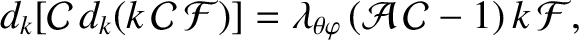(11.160)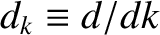, and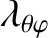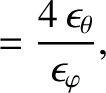(11.161)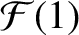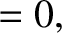(11.162)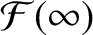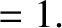(11.163)

Here, the functions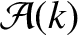,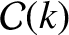,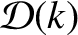, and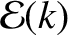are defined in Section 8.11. Note that the factor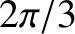in Equation (11.157) is generated by the discontinuity in the function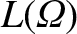(i.e., the discontinuity in the pressure gradient) across the separatrix of the magnetic island chain . If this contribution is omitted then the sign of the integralis reversed.

The values of the first three integrals are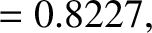(11.164)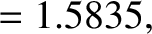(11.165)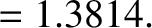(11.166)

Figure 11.1 shows the values of the integralsandas functions of. Note thatandare both only very weak functions of.